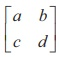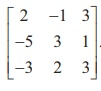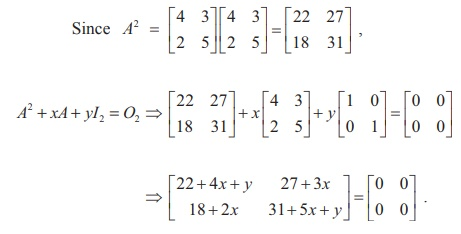Home | | Maths 12th Std | Inverse of a Non-Singular Square Matrix: Solved Example Problems

# Inverse of a Non-Singular Square Matrix: Solved Example Problems

Mathematics : Applications of Matrices and Determinants | Inverse of a Non-Singular Square Matrix: Solved Example Problems

### Example 1.1

If A =, verify that A (adj A) =  (adj A) A = | A | I3 .

SolutionExample 1.2

If A =is non-singular, find AŌłÆ1

Solution

We first find adj A. By definition, we get### Example 1.3

Find the inverse of the matrixSolutionExample 1.4

If is a non-singular matrix of odd order, prove that |adj A| is positive.

Solution

Let A be a non-singular matrix of order 2m + 1 , where m = 0,1, 2, .. . . Then, we get |A| ŌēĀ 0 and, by theorem 1.9 (ii), we have |adj A| = |A|(2m+1)-1 = |A|2m

Since |A|2m is always positive, we get that |adj A| is positive.

Example 1.5

Find a matrix  if adj( ASolutionExample 1.6

If adj =find AŌłÆ1.

SolutionExample1.7

If is symmetric, prove that adj is also symmetric.

Solution

Suppose A is symmetric. Then, AT = A and so, by theorem 1.9 (vi), we get

### Example 1.8

Verify the property  ( AT  )ŌłÆ1  = ( AŌłÆ1 )T  with.

### Solution

For the given A, we get |A |= (2) (7) -  (9)(1) = 14 ŌłÆ 9 = 5 .From (1) and (2), we get (A-1) = (AT)-1. Thus, we have verified the given property.

Example 1.9

Verify ( AB)ŌłÆ1 = BŌłÆ1 AŌłÆ1 withSolutionAs the matrices in (1) and (2) are same, (AB) ŌłÆ1 = B-1 A-1 is verified.

### Example 1.10

If  A  =find and such that A2 + xA + yI2=O2, Hence, find AŌłÆ1.

SolutionSo, we get 22 + 4x + y =0, 31+5x+y=0, 27+3x=0 and 18+2x=0

Hence x = ŌłÆ9 and y =14.Then, we get A2 - 9A + 14I2 = O2

Post-multiplying this equation by AŌłÆ1 , we get A ŌĆō 9I2 + 14A-1 = O2.  Hence, we get### Example 1.11

Prove thatis orthogonal.

### SolutionSimilarly, we get ATA = I2 . Hence AAT = ATA = I2 ŌćÆ A is orthogonal.

### Example 1.12

If A =, is orthogonal, find aand , and hence AŌłÆ1.

### Solution

If A is orthogonal, then AAT = AT A = I3 . So, we haveStudy Material, Lecturing Notes, Assignment, Reference, Wiki description explanation, brief detail
12th Mathematics : UNIT 1 : Applications of Matrices and Determinants : Inverse of a Non-Singular Square Matrix: Solved Example Problems |